Tamil Nadu Board of Secondary EducationSSLC (English Medium) Class 9th
Advertisement Remove all ads

# Samacheer Kalvi solutions for Mathematics Class 9th Tamil Nadu State Board chapter 6 - Trigonometry [Latest edition]

Advertisement Remove all ads

#### Chapters## Chapter 6: Trigonometry

Exercise 6.1Exercise 6.2Exercise 6.3Exercise 6.4Exercise 6.5
Exercise 6.1 [Pages 227 - 228]

### Samacheer Kalvi solutions for Mathematics Class 9th Tamil Nadu State Board Chapter 6 Trigonometry Exercise 6.1 [Pages 227 - 228]

Exercise 6.1 | Q 1 | Page 227

From the given figure, find all the trigonometric ratios of angle B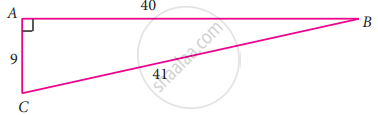Exercise 6.1 | Q 2. (i) | Page 227

From the given figure, find the values of sin B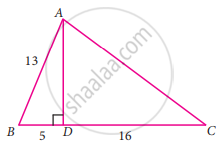Exercise 6.1 | Q 2. (ii) | Page 227

From the given figure, find the values of sec BExercise 6.1 | Q 2. (iii) | Page 227

From the given figure, find the values of cot BExercise 6.1 | Q 2. (iv) | Page 227

From the given figure, find the values of cos CExercise 6.1 | Q 2. (v) | Page 227

From the given figure, find the values of tan CExercise 6.1 | Q 2. (vi) | Page 227

From the given figure, find the values of cosec CExercise 6.1 | Q 3 | Page 227

If 2 cos θ = sqrt(3), then find all the trigonometric ratios of angle θ

Exercise 6.1 | Q 4 | Page 227

If cos A = 3/5, then find the value of (sin"A" - cos"A")/(2tan"A")

Exercise 6.1 | Q 5 | Page 227

If cos A = (2x)/(1 + x^2), then find the values of sin A and tan A in terms of x

Exercise 6.1 | Q 6 | Page 227

If sin θ = "a"/sqrt("a"^2 + "b"^2), then show that b sin θ = a cos θ

Exercise 6.1 | Q 7 | Page 227

If 3 cot A = 2, then find the value of (4sin"A" - 3cos"A")/(2sin"A" + 3cos"A")

Exercise 6.1 | Q 8 | Page 227

If cos θ : sin θ = 1 : 2, then find the value of (8costheta - 2sintheta)/(4costheta + 2sintheta

Exercise 6.1 | Q 9 | Page 227

From the given figure, prove that θ + ∅ = 90°. Also prove that there are two other right angled triangles. Find sin α, cos β and tan ∅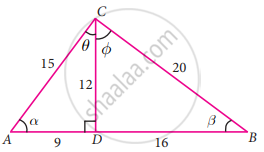Exercise 6.1 | Q 10 | Page 228

A boy standing at a point O finds his kite flying at a point P with distance OP = 25 m. It is at a height of 5 m from the ground. When the thread is extended by 10 m from P, it reaches a point Q. What will be the height QN of the kite from the ground? (use trigonometric ratios)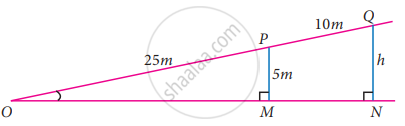Advertisement Remove all ads
Exercise 6.2 [Page 232]

### Samacheer Kalvi solutions for Mathematics Class 9th Tamil Nadu State Board Chapter 6 Trigonometry Exercise 6.2 [Page 232]

Exercise 6.2 | Q 1. (i) | Page 232

Verify the following equalities:

sin2 60° + cos2 60° = 1

Exercise 6.2 | Q 1. (ii) | Page 232

Verify the following equalities:

1 + tan2 30° = sec2 30°

Exercise 6.2 | Q 1. (iii) | Page 232

Verify the following equalities:

cos 90° = 1 – 2sin2 45° = 2cos2 45° – 1

Exercise 6.2 | Q 1. (iv) | Page 232

Verify the following equalities:

sin 30° cos 60° + cos 30° sin 60° = sin 90°

Exercise 6.2 | Q 2. (i) | Page 232

Find the value of the following:

(tan45^circ)/("cosec"30^circ) + (sec60^circ)/(cot45^circ) - (5sin90^circ)/(2cos0^circ)

Exercise 6.2 | Q 2. (ii) | Page 232

Find the value of the following:

(sin 90° + cos 60° + cos 45°) × (sin 30° + cos 0° – cos 45°)

Exercise 6.2 | Q 2. (iii) | Page 232

Find the value of the following:

sin2 30° – 2 cos3 60° + 3 tan4 45°

Exercise 6.2 | Q 3 | Page 232

Verify cos3A = 4cos3A – 3cosA, when A = 30°

Exercise 6.2 | Q 4 | Page 232

Find the value of 8 sin 2x, cos 4x, sin 6x, when x = 15°

Advertisement Remove all ads
Exercise 6.3 [Page 235]

### Samacheer Kalvi solutions for Mathematics Class 9th Tamil Nadu State Board Chapter 6 Trigonometry Exercise 6.3 [Page 235]

Exercise 6.3 | Q 1. (i) | Page 235

Find the value of the following:

((cos 47^circ)/(sin 43^circ))^2 + ((sin 72^circ)/(cos 18^circ))^2 - 2cos^2 45^circ

Exercise 6.3 | Q 1. (ii) | Page 235

Find the value of the following:

(cos 70^circ)/(sin 20^circ) + (cos 59^circ)/(sin31^circ) + cos theta/(sin(90^circ - theta))- 8cos^2 60^circ

Exercise 6.3 | Q 1. (iii) | Page 235

Find the value of the following:

tan 15° tan 30° tan 45° tan 60° tan 75°

Exercise 6.3 | Q 1. (iv) | Page 235

Find the value of the following:

cot theta/(tan(90^circ - theta)) + (cos(90^circ - theta) tantheta sec(90^circ - theta))/(sin(90^circ - theta)cot(90^circ - theta)"cosec"(90^circ - theta))

Advertisement Remove all ads
Exercise 6.4 [Page 239]

### Samacheer Kalvi solutions for Mathematics Class 9th Tamil Nadu State Board Chapter 6 Trigonometry Exercise 6.4 [Page 239]

Exercise 6.4 | Q 1. (i) | Page 239

Find the value of the following:

sin 49°

Exercise 6.4 | Q 1. (ii) | Page 239

Find the value of the following:

cos 74° 39′

Exercise 6.4 | Q 1. (iii) | Page 239

Find the value of the following:

tan 54° 26′

Exercise 6.4 | Q 1. (iv) | Page 239

Find the value of the following:

sin 21° 21′

Exercise 6.4 | Q 1. (v) | Page 239

Find the value of the following:

cos 33° 53′

Exercise 6.4 | Q 1. (vi) | Page 239

Find the value of the following:

tan 70° 17′

Exercise 6.4 | Q 2. (i) | Page 239

Find the value of θ if sin θ = 0.9975

Exercise 6.4 | Q 2. (ii) | Page 239

Find the value of θ if cos θ = 0.6763

Exercise 6.4 | Q 2. (iii) | Page 239

Find the value of θ if tan θ = 0.0720

Exercise 6.4 | Q 2. (iv) | Page 239

Find the value of θ if cos θ = 0.0410

Exercise 6.4 | Q 2. (v) | Page 239

Find the value of θ if tan θ = 7.5958

Exercise 6.4 | Q 3. (i) | Page 239

Find the value of the following:

sin 65° 39′ + cos 24° 57’ + tan 10° 10′

Exercise 6.4 | Q 3. (ii) | Page 239

Find the value of the following:

tan 70° 58′ + cos 15° 26′ – sin 84° 59′

Exercise 6.4 | Q 4 | Page 239

Find the area of a right triangle whose hypotenuse is 10 cm and one of the acute angle is 24°24′

Exercise 6.4 | Q 5 | Page 239

Find the angle made by a ladder of length 5m with the ground, if one of its end is 4m away from the wall and the other end is on the wall

Exercise 6.4 | Q 6 | Page 239

In the given figure, HT shows the height of a tree standing vertically. From a point P, the angle of elevation of the top of the tree (that is ∠P) measures 42° and the distance to the tree is 60 metres. Find the height of the tree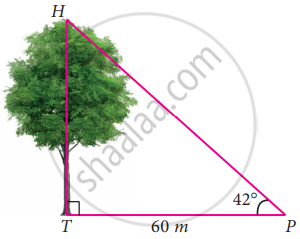Advertisement Remove all ads
Exercise 6.5 [Pages 239 - 240]

### Samacheer Kalvi solutions for Mathematics Class 9th Tamil Nadu State Board Chapter 6 Trigonometry Exercise 6.5 [Pages 239 - 240]

#### Multiple choice questions

Exercise 6.5 | Q 1 | Page 239

If sin 30° = x and cos 60° = y, then x2 + y2 is

• 1/2

• 0

• sin 90°

• cos 90°

Exercise 6.5 | Q 2 | Page 239

If tan θ = cot 37°, then the value of θ is

• 37°

• 53°

• 90°

Exercise 6.5 | Q 3 | Page 239

The value of tan 72° tan 18° is

• 0

• 1

• 18°

• 72°

Exercise 6.5 | Q 4 | Page 239

The value of (2tan30^circ)/(1 - tan^2 30^circ) is equal to

• cos 60°

• sin 60°

• tan 60°

• sin 30°

Exercise 6.5 | Q 5 | Page 240

If 2 sin 2θ = sqrt(3) then the value of θ is

• 90°

• 30°

• 45°

• 60°

Exercise 6.5 | Q 6 | Page 240

The value of 3 sin 70° sec 20° + 2 sin 49° sec 51° is

• 2

• 3

• 5

• 6

Exercise 6.5 | Q 7 | Page 240

The value of (1 - tan^2 45^circ)/(1 + tan^2 45^circ) is

• 2

• 1

• 0

• 1/2

Exercise 6.5 | Q 8 | Page 240

The value of cosec(70° + θ) – sec(20° − θ) + tan(65° + θ) – cot(25° − θ) is

• 0

• 1

• 2

• 3

Exercise 6.5 | Q 9 | Page 240

The value of tan 1° tan 2° tan 3°…. tan 89° is

• 0

• 1

• 2

• sqrt(3)/2

Exercise 6.5 | Q 10 | Page 240

Given that sin α = 1/2 and cos β = 1/2, then the value of α + β is

• 90°

• 30°

• 60°

Advertisement Remove all ads

## Chapter 6: Trigonometry

Exercise 6.1Exercise 6.2Exercise 6.3Exercise 6.4Exercise 6.5## Samacheer Kalvi solutions for Mathematics Class 9th Tamil Nadu State Board chapter 6 - Trigonometry

Samacheer Kalvi solutions for Mathematics Class 9th Tamil Nadu State Board chapter 6 (Trigonometry) include all questions with solution and detail explanation. This will clear students doubts about any question and improve application skills while preparing for board exams. The detailed, step-by-step solutions will help you understand the concepts better and clear your confusions, if any. Shaalaa.com has the Tamil Nadu Board of Secondary Education Mathematics Class 9th Tamil Nadu State Board solutions in a manner that help students grasp basic concepts better and faster.

Further, we at Shaalaa.com provide such solutions so that students can prepare for written exams. Samacheer Kalvi textbook solutions can be a core help for self-study and acts as a perfect self-help guidance for students.

Concepts covered in Mathematics Class 9th Tamil Nadu State Board chapter 6 Trigonometry are Trigonometry, Trigonometric Ratios of Complementary Angles, Trigonometric Ratios and Its Reciprocal, Reciprocal Relation Between Trigonometric Ratios, Trigonometric Ratios of Some Special Angles, Method of Using Trigonometric Table.

Using Samacheer Kalvi Class 9th solutions Trigonometry exercise by students are an easy way to prepare for the exams, as they involve solutions arranged chapter-wise also page wise. The questions involved in Samacheer Kalvi Solutions are important questions that can be asked in the final exam. Maximum students of Tamil Nadu Board of Secondary Education Class 9th prefer Samacheer Kalvi Textbook Solutions to score more in exam.

Get the free view of chapter 6 Trigonometry Class 9th extra questions for Mathematics Class 9th Tamil Nadu State Board and can use Shaalaa.com to keep it handy for your exam preparation

Advertisement Remove all ads
Share
Notifications

View all notifications

Forgot password?